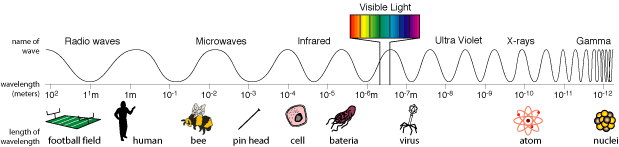ps://c328740.ssl.cf1.rackcdn.com/mathjax/latest/MathJax.js?config=TeX-AMS-MML_HTMLorMML">

# Analyzing the Universe - Course Wiki: The Nature of Light

## Composition of Light: The Electromagnetic Spectrum

Sir Isaac Newton discovered that the white light produced by the sun separated into the colors of the rainbow when it passed through a prism. In order to demonstrate that it was not the prism itself distorting the light, Newton focused light of one particular color of the rainbow formed by a first prism into yet another prism, and the light that exited the prism was the same color of the light which entered it.

As it turns out, the human eye sees but a narrow band of light in electromagnetic spectrum (depicted in the cartoon below with wavelength comparison to some length-scales of commonly known objects). Goddard Space Flight Center's education page on the Electromagnetic Spectrum, seen in the iframe below, (you must really read this!) does an exemplary job in breaking down the various regimes of light, and the devices we create to overcome the limitations of our own senses.

##### (If you have difficulties seeing the iframe or it is blank, click here.)

The page also explains why we need space telescopes for various regimes in this electromagnetic spectrum. This relates especially to our class since we are Analyzing the Universe in the X-ray regime, and in particular, using the Chandra Space Observatory that currently orbits our earth 139,000 km (86,500 mi) above its surface (in comparison the atmosphere has a thickness of roughly 100 km (62 mi)).## A Universal Constant: The Speed of Light

Throughout our universe, all photons appear to travel at a constant velocity in a given material. For a vacuum, James Clerk Maxwell showed that the speed of light, c, is about 300 million meters per second (186,000 miles/second). This speed remains constant independent of the reference frame of the observer. This is counter-intuitive to our everyday experience though.

As a practical example, imagine we are driving our car at a speed of of 60 miles per hour on the highway. Objects that are stationary on the ground will appear to be flying behind us at a speed of 60 miles per hour. Also, if we pass a car traveling in the same direction it will appear to move backwards in our frame of reference. Furthermore, if a car approaches us head-on at a speed of 60 miles per hour, it will appear to fly by us at a speed of 120 miles per hour. But light is not like this at all! If you are approaching a photon near the speed of light, it will fly by you at the speed of light. Also, if you are traveling very close to the speed of light and shoot photons ahead of you, they will appear to leave the car once again at the speed of light. Albert Einstein spelled all of this out eloquently in his Theory of Special Relativity. It turns out that the very nature of space and time itself is quite different than we ordinarily thought.

Earlier I stated that the speed of light for a given material remains constant. This implies then that the speed of light can take on different values in different media, and that is exactly what occurs. For example the speed of light in fibre optic cables is approximately 23 c. The ratio of the speed of light in a vacuum to the speed of light in a material "i" gives us the index of refraction of said material "i". We commonly represent the index of refraction, $n_i$ as,

$$n_i=\frac{\rm{c}}{{\rm{c}}_i},\:\:\:\left(1\right)$$

where $\rm{c}_i$ is the local speed of light in material "i".

## The Photon: A Particle and A Wave

Besides acting as a wave, a photon can also behave like a particle. This is best demonstrated by the Photoelectric Effect, where photons hit electrons in a material and can cause them to be ejected if the individual photon has more energy then the binding energy of the electron that usually keeps them orbiting the nucleus in a stable fashion. Another demonstration that photons behave as discrete particles is the existence of atomic spectra that result from excitations of the electrons of an atom, which only occur at very specific (quantized) energies of photons.

On the other hand, the double-slit experiment is the classic experiment that shows how a photon behaves as though it were wave, and is perhaps best described by the silky voice of Morgan Freeman in the video found here. Thus, light can be described as a discrete bundle or chunk (quantum) of energy, and as a wave with an unique wavelength or frequency.

The Energy of a photon (γ) is defined as,

$$E_{\gamma}=\rm{h}\;\nu\,,\:\:\:\left(2\right)$$

where ν is the frequency of the photon, or the number of peaks in the wave pass a given point per second, and h is Planck's Constant, which is roughly 7 ×10-34 Joule•Seconds, where Joule•Seconds are the same units as angular momentum, and (among other things) sets a limit for the minimum amount of energy any sub-atomic particle can have. A photon also exhibits an implicit relationship between its speed, wavelength, and frequency that goes as,

$$\rm{c}=\lambda\;\nu\,,\:\:\:\left(3\right)$$

where c is the speed of light in a given medium, and λ is the wavelength of the light (the distance between peaks in the wave). We can then recast equation (2) in terms of wavelength instead of frequency of the light wave as,

$$E_{\gamma}=\frac{\rm{h}\;\rm{c}}{\lambda}\,.\:\:\:\left(4\right)$$

Note that Planck's constant h, can be recast in different unit systems based on the desired energy units, for example, electron-volt seconds, where now, h = 4 × 10-15 eV•Seconds. Moreover, we are free to use any unit of wavelength as long as the speed of light's length dimension is consistent. For instance if you wanted to express wavelength in units of centimeters, you would need to define the speed of light to be, c = 3 × 1010 cm/s.